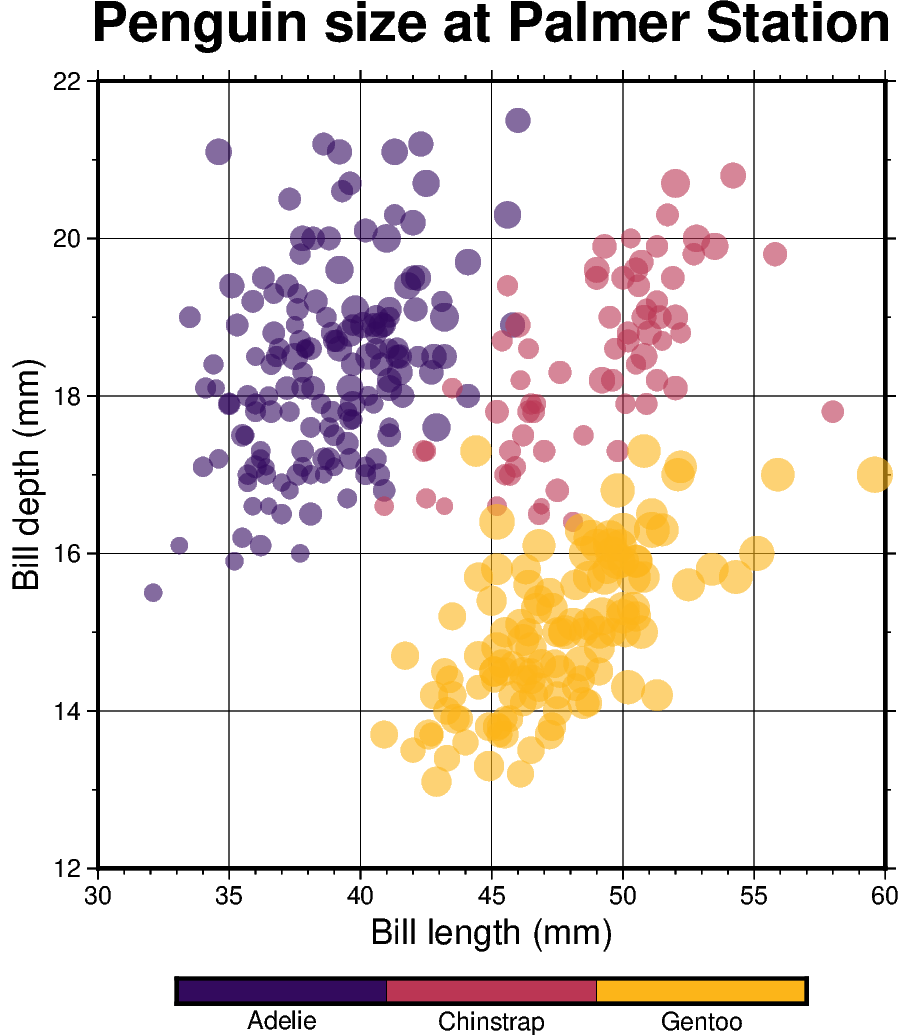# Color points by categories¶

The `pygmt.Figure.plot` method can be used to plot symbols which are color-coded by categories. In the example below, we show how the Palmer Penguins dataset can be visualized. Here, we can pass the individual categories included in the “species” column directly to the `color` parameter via `color=df.species.cat.codes.astype(int)`. Additionally, we have to set `cmap=True`. A desired colormap can be selected via the `pygmt.makecpt` method.Out:

```<IPython.core.display.Image object>
```

```import pandas as pd
import pygmt

# Load sample penguins data and convert 'species' column to categorical dtype
df.species = df.species.astype(dtype="category")

# Use pygmt.info to get region bounds (xmin, xmax, ymin, ymax)
# The below example will return a numpy array like [30.0, 60.0, 12.0, 22.0]
region = pygmt.info(
table=df[["bill_length_mm", "bill_depth_mm"]],  # x and y columns
per_column=True,  # report the min/max values per column as a numpy array
# round the min/max values of the first two columns to the nearest multiple
# of 3 and 2, respectively
spacing=(3, 2),
)

# Make a 2D categorical scatter plot, coloring each of the 3 species differently
fig = pygmt.Figure()

# Generate a basemap of 10 cm x 10 cm size
fig.basemap(
region=region,
projection="X10c/10c",
frame=[
'xafg+l"Bill length (mm)"',
'yafg+l"Bill depth (mm)"',
'WSen+t"Penguin size at Palmer Station"',
],
)

# Define a colormap to be used for three categories, define the range of the
# new discrete CPT using series=(lowest_value, highest_value, interval),
# use color_model="+cAdelie,Chinstrap,Gentoo" to write the discrete color palette
# "inferno" in categorical format and add the species names as annotations for the
# colorbar

fig.plot(
# Use bill length and bill depth as x and y data input, respectively
x=df.bill_length_mm,
y=df.bill_depth_mm,
# Vary each symbol size according to another feature (body mass, scaled by 7.5*10e-5)
size=df.body_mass_g * 7.5e-5,
# Points colored by categorical number code
color=df.species.cat.codes.astype(int),
# Use colormap created by makecpt
cmap=True,
# Do not clip symbols that fall close to the map bounds
no_clip=True,
# Use circles as symbols with size in centimeter units
style="cc",
# Set transparency level for all symbols to deal with overplotting
transparency=40,
)